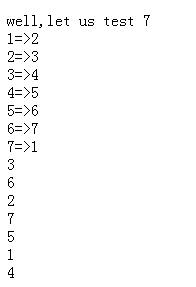# PHP基于关联数组20行代码搞定约瑟夫问题示例

ps：其实个人挺反感用代码行数来衡量代码数量的，感觉常把代码行数挂嘴边的大多无奈装2。此文仅属闲余娱乐。

```<?php
\$n=7;
echo "well,let us test \$n<br/>";
for(\$i=1;\$i<\$n;\$i++){
\$a["\$i"]=\$i+1;
\$flag[\$i]="in";
}
\$a["\$n"]=1;
foreach(\$a as \$key=>\$value){
echo \$key."=>".\$value."</br>";
}
\$key=1;\$out=0;\$r=0;
while(\$out<\$n){
\$r++;
if(\$r==2){
echo"\$a[\$key]<br/>";// out
\$a[\$key]=\$a[\$a[\$key]];
\$out++;\$r=0;
}
\$key=\$a[\$key];
}

```php基于环形链表解决约瑟夫环问题示例

PHP简单实现循环链表功能示例

PHP根据树的前序遍历和中序遍历构造树并输出后序遍历的方法

Copyright © 2010 Gimoo.Net. All Rights Rreserved  京ICP备05050695号